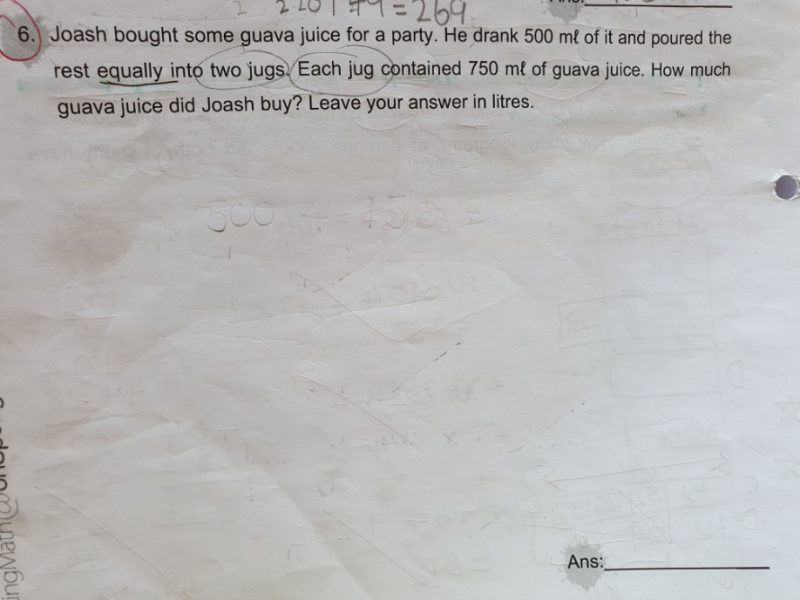# QuestionSource: nil

Working backwards,
750 ml x 2 = 1500 ml
1500 ml + 500 ml = 2000 ml = 2 l

Ans : 2 l.

0 Replies 0 Likes

Understand this: if Joash drank 500ml of the guava juice.

Can you find the remainder? (What is left after he drank 500ml of the guava juice)

Yes, right?

Remainder => 750ml + 750ml

= 1500ml

Remainder => 750ml x 2

= 1500ml

You found the remainder, what is next? You add up what Joash had drunk with the remainder, right?

Bought => 500ml + 1500ml

= 2000ml

Now we have to change it to litres.

2000ml/1000 = 2 litres

But why divide it by 1000? Litres is 1000ml, right?

So two 2000ml is equal to 2 litres.

So that is question solved.

0 Replies 1 Like